# Example 2: Reinforcement design of a beam section with rebar size constraints

Watch the video of this example >

###### Data for Cross Section Analysis & Design application

In this example, we are going to see how rebar size constraints can be used for designing reinforced concrete cross sections, so that the calculated rebars have a predefined area proportion, as shown in the picture below. The used cross section is similar to that defined in Example 1.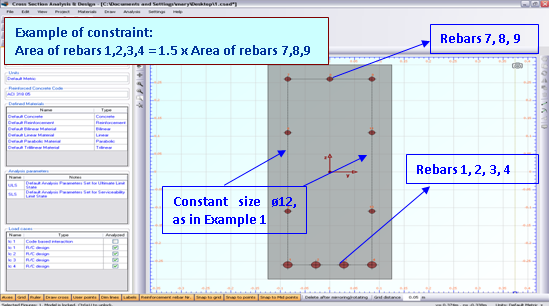Demonstration of area constraint between rebars

Let’s assume the following constraint: The area of each rebar 1, 2, 3 and 4 to the area of 7, 8 or 9 should be equal to 1.5. We suppose that side rebars are constant ø12, as in Example 1.

Same to these defined in Example 1.

#### Opening a file from disk

First of all we click on the File menu and select Open in order to open the file we created in Example 1.

#### Unlock the model

Afterwards, if the model is locked, we click on Edit -> Unlock model, in order to modify the geometry of the cross section.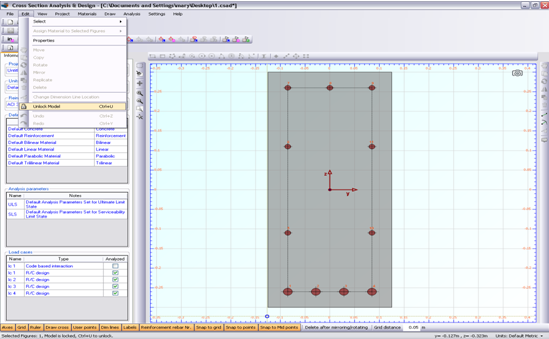In the popup window, we choose Yes to unlock the model.#### Application of constraints

Next, we move the mouse over the reinforcement line at the top rebars (7, 8, 9) till the “rebar line” text appears.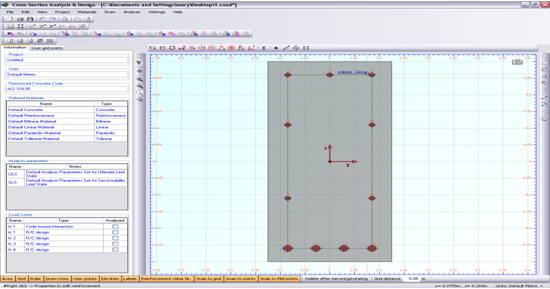After right-clicking, we select “Properties” from the menu, in order to view the properties of the top rebars.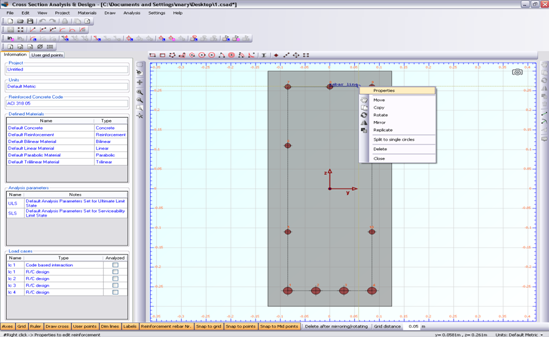In the corresponding form, we make sure that the option “Constant size for design” is unchecked and the “Grow factor” value is set to 1 and finally click OK.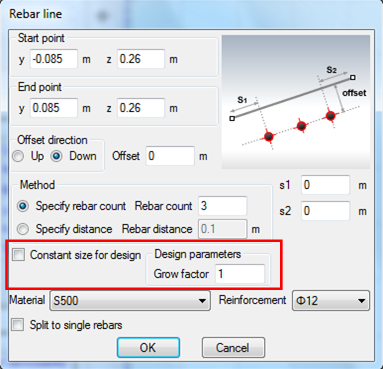Proceeding respectively for the bottom rebars, we set the “Grow factor” value to 1.5.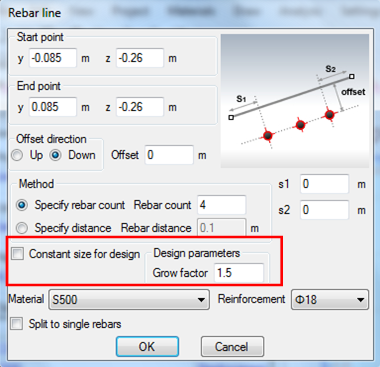Note that the selected Reinforcement values are not important, since the reinforcement bars will be designed by the program.

#### Carry out the design

We just click Analysis -> Reinforcement design -> Analyze, to perform the reinforcement design procedure, as in Example 1.

The program asks if it should assign the calculated rebars to the existing ones. We click on “Yes”.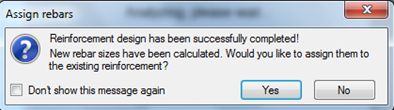#### Results

The results can be obtained from the Analysis -> Reinforcement design -> Show results menu item.

As we can see, the cross section is adequate and the bottom rebars have been chosen to be ø18. Top rebars are now of size ø14. But the calculated required area for reinforcement bars 1 to 4 and 7 to 9 conforms to the applied constraint, since 2.2966 cm2 = 1.5 x 1.5311 cm2.

Remaining rebars (side reinforcement) did not change (ø12), as their “Constant size for design” option has been checked (in Example 1).

Note: The program has calculated the required rebar sizes so that the provided reinforcement ratio lies between the limits specified in the Reinforced Concrete Regulation form and also the applied constraint is valid. Furthermore, it should be noticed, that the constraint may not be precisely valid for the placed rebar areas as well, since the program chooses rebars from the available ones in project (Project -> Available Rebars menu item) under the condition that their area is greater than the required one.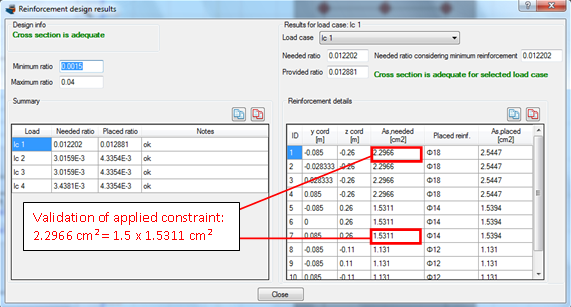## Watch the video of this exampleHave any questions? Do not hesitate to contact us! Contact  us ›